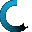Retrieving data ...
Please do not click anywhere else.

If you see no response after 2-3 minutes, then click on the [X] button in the upper right corner to close the window and retry the operation at a later time.
Interactive Multimedia Learning & Teaching Management System Suite## Course Contents

Course Name: Elementary and Middle School Multiplication - Online
• Elementary and Middle School Multiplication
•  1.1 Introduction to Multiplication
•  1.2 Multiplication in Relation to Addition
•  1.3 Multiplication Using Objects
•  1.4 Multiplication Using a Multiplication Table
•  1.5 Multiplication Tricks
•  1.6 Multiplying a 2-digit Number by a 1-digit Number
•  1.7 Multiplying a 3-digit Number and a 1-digit Number
•  1.8 Multiplying a 2-digit Number and a 2-digit Number
•  1.9 Multiplying a 3-digit Number and a 2-digit Number
•  1.10 Multiplying a 4-digit Number and a 2-digit Number
•  1.11 Multiplying a 5-digit Number and a 2-digit Number
•  1.12 Multiplying a 3-digit Number and a 3-digit Number
•  1.13 Multiplication with Three Numbers